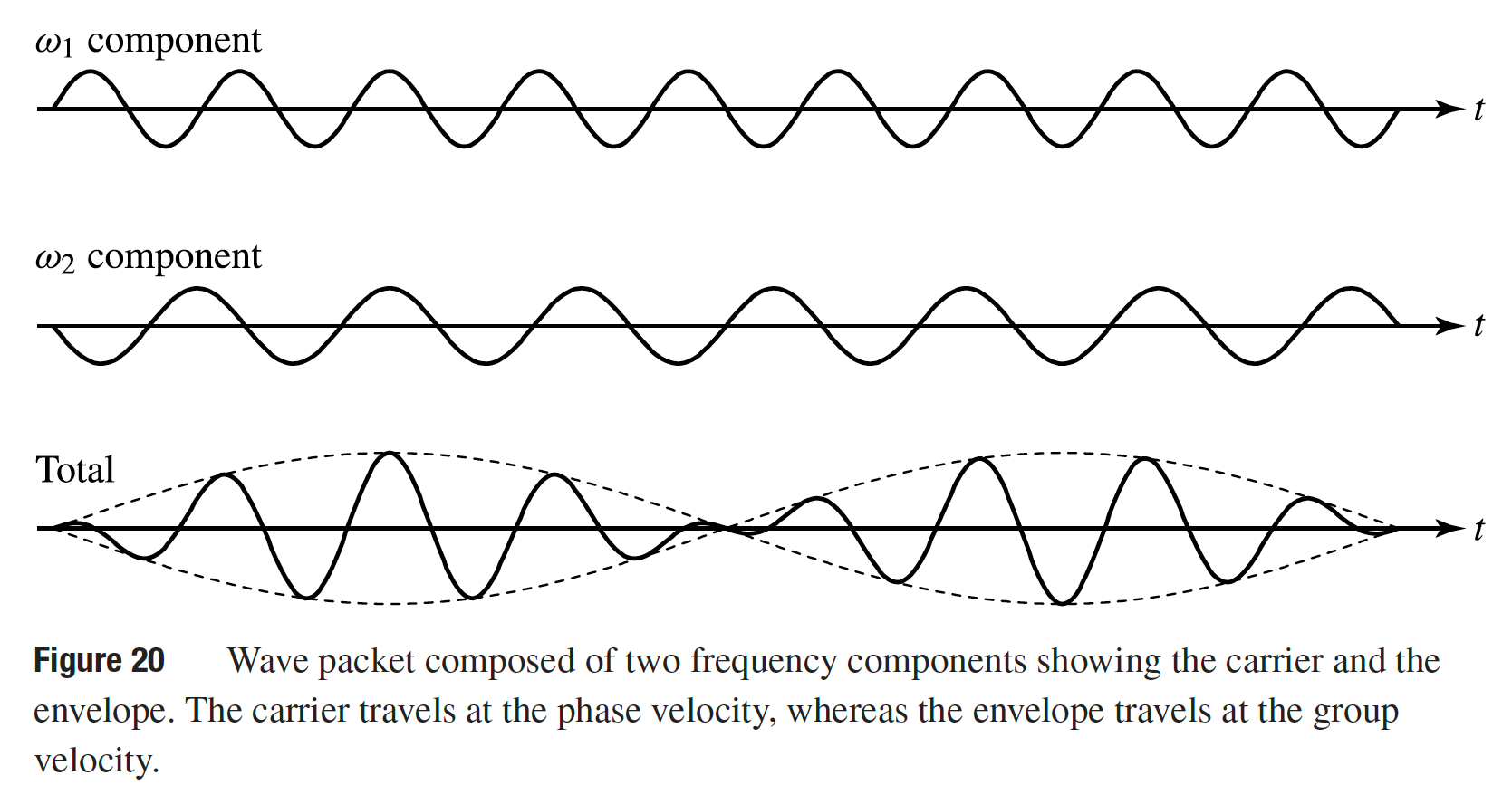# Phase Velocity, Group Velocity, and Dispersion

This is a continuation from the previous tutorial - Reflection and Refraction.

For a monochromatic plane optical wave traveling in the z direction, the electric field can be written as

$\tag{159}\mathbf{E}=\pmb{\mathcal{E}}\exp(\text{i}kz-\text{i}\omega{t})$

where $$\pmb{\mathcal{E}}$$ is a constant vector independent of space and time. This represents a sinusoidal wave whose phase varies with z and t as

$\tag{160}φ=kz-\omega{t}$

For a point of constant phase on the space- and time-varying field, φ = constant and thus kdz - ωdt = 0. If we track this point of constant phase, we find that it is moving with a velocity of

$\tag{161}\nu_p=\frac{\text{d}z}{\text{d}t}=\frac{\omega}{k}$

This is called the phase velocity of the wave. Note that the phase velocity is a function of optical frequency because the refractive index of a medium is a function of frequency. There is phase-velocity dispersion due to the fact that dn/dω ≠ 0. In the case of normal dispersiondn/dω > 0 and dn/dλ < 0; in the case of anomalous dispersiondn/dω < 0 and dn/dλ > 0.In real circumstances, a propagating optical wave rarely contains only one frequency. It usually consists of many frequency components grouped around some center frequency ω0. For the simplicity of illustration, we consider a wave packet traveling in the z direction that is composed of two plane waves of equal real amplitude $$\pmb{\mathcal{E}}$$. The frequencies and propagation constants of the two component plane waves are

\tag{162}\begin{align}\omega_1=\omega_0+\text{d}\omega,\;k_1=k_0+\text{d}k\\\omega_2=\omega_0-\text{d}\omega,\;k_2=k_0-\text{d}k \end{align}

The space- and time-dependent total real field of the wave packet is then given by

\tag{163}\begin{align}\pmb{E}&=\pmb{\mathcal{E}}\exp(\text{i}k_1z-\text{i}\omega_1t)+\text{c.c.}+\pmb{\mathcal{E}}\exp(\text{i}k_2z-\text{i}\omega_2t)+\text{c.c.}\\&=2\pmb{\mathcal{E}}\lbrace\cos[(k_0+\text{d}k)z-(\omega_0+\text{d}\omega)t]+\cos[(k_0-\text{d}k)z-(\omega_0-\text{d}\omega)t]\rbrace\\&=4\pmb{\mathcal{E}}\cos(\text{d}kz-\text{d}\omega{t})\cos(k_0z-\omega_0t)\end{align}

We find that the resultant wave packet has a carrier, which has a frequency ω0 and a propagation constant k0, and an envelope, which varies in space and time as cos(dkz - dωt). This is illustrated in figure 20 above. Therefore, a fixed point on the envelope is defined by dkz - dωt = constant, and it travels with a velocity of

$\tag{164}\nu_g=\frac{\text{d}z}{\text{d}t}=\frac{\text{d}\omega}{\text{d}k}$

This is the velocity of the wave packet and is called the group velocity. Because the energy of a harmonic wave is proportional to the square of its field amplitude, the energy carried by a wave packet that is composed of many frequency components is concentrated in regions where the amplitude of the envelope is large. Therefore, the energy in a wave packet is transported at group velocity vg.

Conclusion:

The constant-phase wavefront travels at the phase velocity, but the group velocity is the velocity at which energy and information travel.

In reality, group velocity is usually a function of optical frequency. Then,

$\tag{165}\frac{\text{d}^2k}{\text{d}\omega^2}=\frac{\text{d}}{\text{d}\omega}\nu_g^{-1}\ne0$

Therefore, d2k/dω2 represents group-velocity dispersion. A dimensionless coefficient for group-velocity dispersion can be defined as

$\tag{166}D=c\omega\frac{\text{d}^2k}{\text{d}\omega^2}=\frac{2\pi{c^2}}{\lambda}\frac{\text{d}^2k}{\text{d}\omega^2}$

Group-velocity dispersion is an important consideration in the propagation of optical pulses. It can cause broadening of an individual pulse, as well as changes in the time delay between pulses of different frequencies.

The sign of the group-velocity dispersion can be either positive or negative. In the case of positive group-velocity dispersiond2k/dω2 >0 and D > 0, a long-wavelength, or low-frequency, pulse travels faster than a short-wavelength, or high-frequency, pulse.

In contrast, a short-wavelength pulse travels faster than a long-wavelength pulse in the case of negative group-velocity dispersiond2k/dω2 < 0 and D < 0. In a given material, the sign of D generally depends on the spectral region of concern.

Group-velocity dispersion and phase-velocity dispersion have different meanings. They should not be confused with each other.

When measuring the transmission delay or the broadening of optical pulses due to dispersion in optical fibers, another dispersion coefficient defined as

$\tag{167}D_\lambda=-\frac{2\pi{c}}{\lambda^2}\frac{\text{d}^2k}{\text{d}\omega^2}=-\frac{D}{c\lambda}$

is usually used. This coefficient is generally expressed as a function of wavelength in units of picoseconds per kilometer per nanometer. It is a direct measure of the chromatic pulse transmission delay over a unit transmission length.

In general, both ε(ω) and n(ω) in an optical medium are frequency dependent, and the propagation constant is

$\tag{168}k=\frac{\omega}{c}n(\omega)$

Therefore, we have

$\tag{169}\nu_p=\frac{c}{n}$

and

$\tag{170}\nu_g=\frac{c}{N}$

where

$\tag{171}N=n+\omega\frac{\text{d}n}{\text{d}\omega}=n-\lambda\frac{\text{d}n}{\text{d}\lambda}$

is called the group index

Using (166) and (167), we also have

$\tag{172}D(\lambda)=\lambda^2\frac{\text{d}^2n}{\text{d}\lambda^2}$

and

$\tag{173}D_\lambda(\lambda)=-\frac{\lambda}{c}\frac{\text{d}^2n}{\text{d}\lambda^2}$

respectively.

Example

The index of refraction of a certain type of glass as a function of optical wavelength around λ = 1.3 μm can be approximated as n = 1.465 - 0.0114(λ - 1.3) - 0.004(λ - 1.3)3, where λ is measured in micrometers. Therefore,

$\frac{\text{d}n}{\text{d}\lambda}=-0.0114-0.012(\lambda-1.3)^2$

$N=n-\lambda\frac{\text{d}n}{\text{d}\lambda}=1.48+0.0156(\lambda-1.3)^2+0.008(\lambda-1.3)^2$

$D=\lambda^2\frac{\text{d}^2n}{\text{d}\lambda^2}=-0.024\lambda^2(\lambda-1.3)$

We find that, in this spectral region, dn/dλ < 0 for any wavelength but D > 0 for λ < 1.3 μm and D < 0 for λ > 1.3 μm. Clearly, this glass has normal phase-velocity dispersion in the entire spectral region around λ < 1.3 μm, but it has positive group-velocity dispersion for λ < 1.3 μm and negative group-velocity dispersion for λ > 1.3 μm. As an example, we find that n ≈ 1.469, N ≈ 1.481, and D ≈ 0.0072 at λ = 1 μm. We also find that n ≈ 1.463, N ≈ 1.481, and D ≈ -0.0108 at λ = 1.5 μm. Because of normal phase-velocity dispersion, the group index is always larger than the refractive index, N > n, in this spectral region.

The next part continues with the Material Dispersion tutorial.